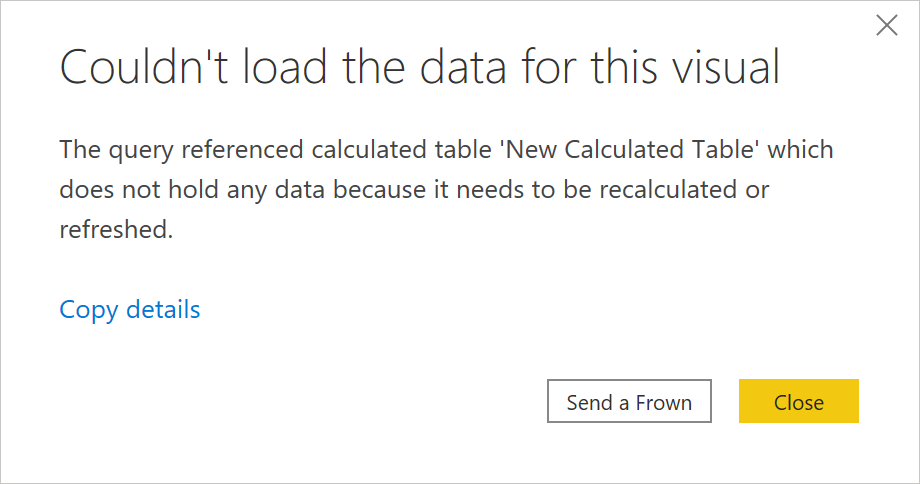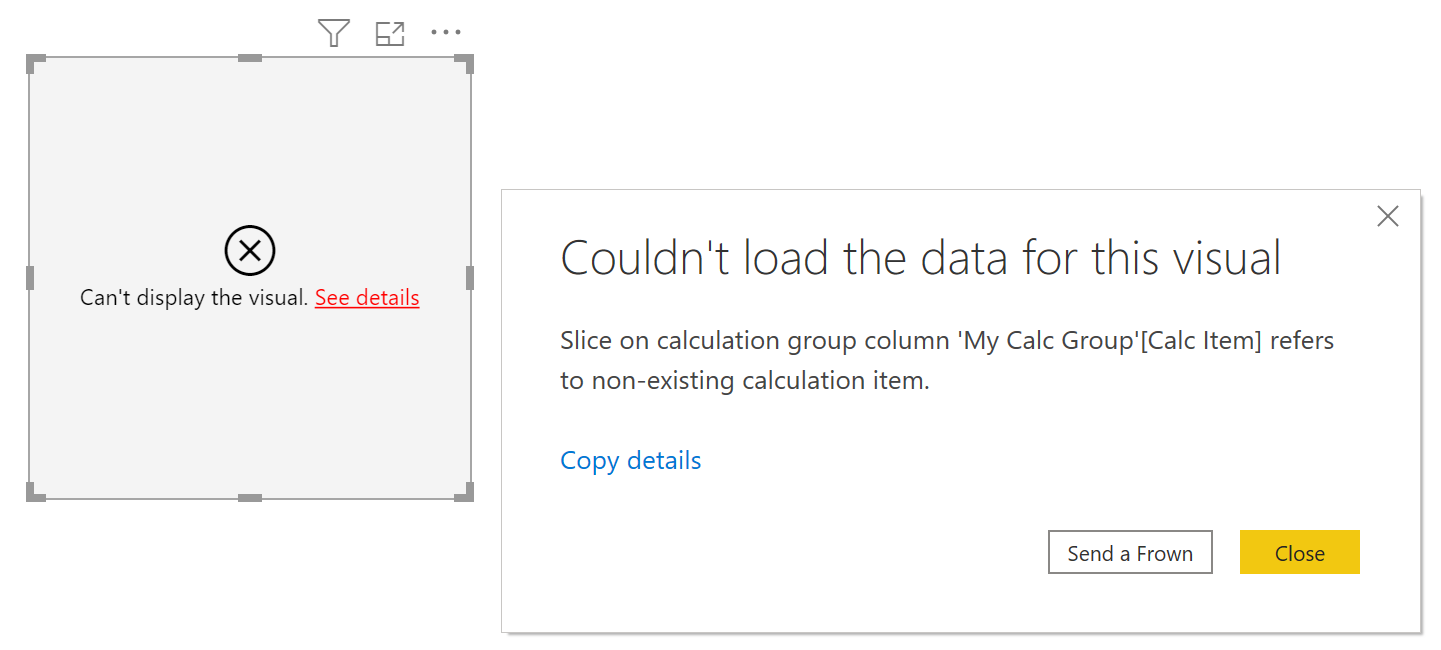# Understanding model recalculation in Power BIHave you ever wondered why in Power BI calculated columns and calculated tables are called calculated and measures are not? That’s because in a certain sense, measures aren’t calculated, and calling them “calculated measures” is wrong. In this blog post, I’m discussing the implications of it–what recalculation in Power BI is, and how you can perform it.

Note: in this blog post, I’m discussing editing Power BI datasets by using the XMLA endpoint, which requires Power BI Premium and may be different from editing local files once this functionality is released. AND if you don’t have Power BI Premium, there’s a way around it–see the link at the end of this blog post.

### What’s “calculated”?

When you create a calculated column or a calculated table In Power BI Desktop, their contents can be viewed immediately in the Data view. They are also recalculated every time you refresh data.

While it may seem counter-intuitive, calculated columns and calculated tables are also recalculated every time you create or edit measures in Power BI Desktop. This is why sometimes in large models editing a measure takes a long time, accompanied by the “Working on it” message:How do you know it’s true? You can perform a simple test:

1. Create a calculated column by using the following formula:
`RightNow = NOW()`
2. Go to the Data view to see the result and note the time.
3. Create or edit any measure.
4. Note that the time in the RightNow column has been updated.

Also, if you close and open the file, the RightNow column will show different time too–Power BI Desktop recalculates the model very often, as you can see.

Measures are different from columns and tables: in Power BI Desktop, you don’t see what they return until you use them in a visual, and they don’t need to be calculated in advance, hence they’re measures, not calculated measures. So why am I so pedantic about it?

### Editing Power BI datasets by using external tools

When you’re editing a Power BI dataset by using an external tool, like Tabular Editor, your model is not automatically recalculated every time you make a change to it. This is not an issue when you only edit measures, because measures aren’t calculated, and you can use them right after you save your changes. The situation is different with calculated columns, calculated tables, and calculation groups.

Tabular Editor only deploys metadata, and it doesn’t process anything. The implication of it is if you create a calculated object–a calculation group, for example–you won’t be able to use it immediately in a report, and you’ll get the following error:Slice on calculation group column ‘My Calc Group'[Calc Item] refers to non-existing calculation item.What does it mean? It means our calculation group isn’t ready to be used. Why? Because it’s not processed yet. Not the most useful error message, I might quickly add. The error message shown when you try to use a newly created calculated table (in the beginning of this blog post) at least says what needs to be done 🙂

### Recalculating your model

If you can’t or don’t want to refresh your dataset in Power BI service, you can recalculate it.

##### Using SSMS

One way of doing it is to use SQL Server Management Studio:

1. Connect to your Power BI workspace.
2. Right-click a dataset and select Process Database.
3. Select Process Recalc from the Mode drop-down list.
4. Select OK.

This will recalculate your model, and you’ll be able to use your calculated objects.

##### Using DAX Studio

What if you don’t want to install another tool just to recalculate your model? You can do it from DAX Studio! I’d guess that there are a lot of Power BI people who use DAX Studio but not SSMS.

Connect to your workspace in DAX Studio, replace the yellow bit with your dataset name, and run the following code:

``````{
"refresh": {
"type": "calculate",
"objects": [
{
"database": "YOUR DATASET NAME"
}
]
}
}``````

You’ll see the following result in the Output section:Despite this looking like an error, we’ve got what we wanted — the dataset is recalculated, and now we can use our calculated objects!